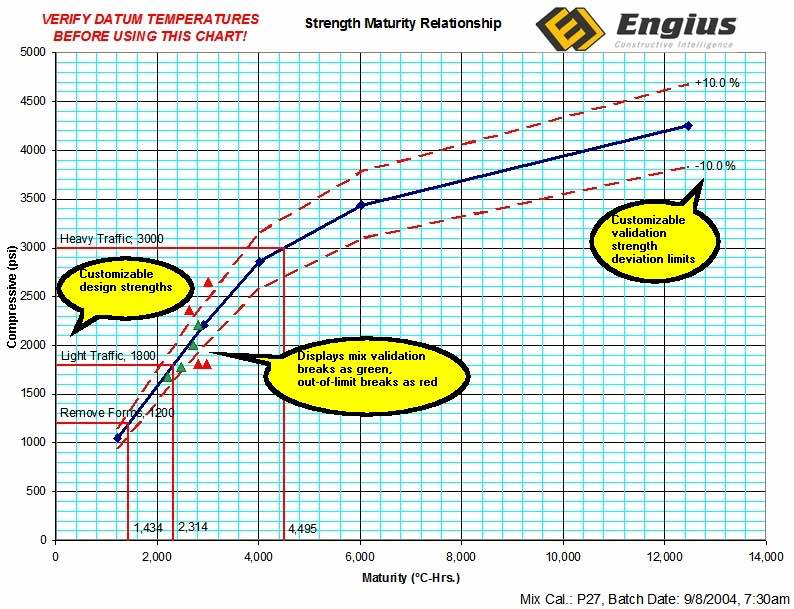Feb 26, 2016

Feb 26, 2016

Feb 26, 2016

# How to Determine Maturity of ConcreteOne of the methods to determine the strength of concrete is to find maturity of concrete. Maturity of concrete is defined as Summation of product of age and temperature (Curing).

Concrete Maturity = ∑ (Time x Temperature)

Hydration can take place at minimum of -10°C, below this water crystals (ice) do not react with cement.

Datum Temperature = -10°C

If Day Temperature = 15°C then

Curing temperature = 15-(-10)=25°C

Maturity of concrete after 28 days kept at 25°C is given as

Maturity = 28 x 24 x (25-(-10)) = 23520°C hr.

Concrete is fully matured when it is cured at 18°C till 28 days. For ordinary concrete maturity should not be less than 19800°C hr

 Strength A B <17.5 MPa 10 70 17.5-35 MPa 21 61 35-52.5 MPa 32 52 52.5-70 MPa 43 43

## (a) Data to be collected:

1. Fineness modulus of selected F.A.
2. Unit weight of dry coarse aggregate.
3. Sp. gravity of coarse and fine aggregates in SSD condition
4. Absorption characteristics of both coarse and fine aggregates.
5. Specific gravity of cement.

## (b) From the minimum strength specified, estimate the average design strength f 'CR either by using standard deviation or by using coefficient of variation.

• 30 Consecutive Tests (1 Test = Ave of 2 Cylinders)
• Determine S= Standard Deviation of Tests
• f 'CR = f 'C + 1.34 S
• f 'CR = f 'C + 2.33 S – 500 (Take Larger Value)

WHERE:

f 'C = Specified Design Concrete Strength
f 'CR = Required Average Compressive Strength Used for Selecting Concrete Proportions